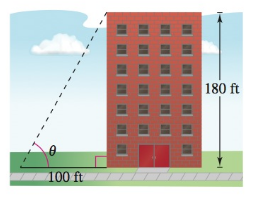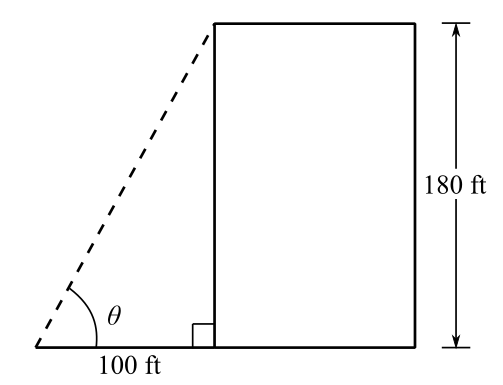Chapter 8.2, Problem 78E### Calculus: An Applied Approach (Min...

10th Edition
Ron Larson
ISBN: 9781305860919

#### Solutions

Chapter
Section### Calculus: An Applied Approach (Min...

10th Edition
Ron Larson
ISBN: 9781305860919
Textbook Problem
1 views

# Height The height of a building is 180 feet. Find the angle of elevation (in degrees) to the top of the building from a point 100 feet from the base of the building (see figure).To determine

To calculate: The angle of elevation θ when the height of a building is 180 feet and distance between a point and base of building is 100 feet.Explanation

Given Information:

The figure is shown below,

Formula used:

The definition of tangent,

tanθ=yx

Where, x is the length of the side adjacent to θ, y is the length of the side opposite to θ, and r=x2+y2 is the length of hypotenuse which is not equal to 0.

Calculation:

Consider the provided figure,

### Still sussing out bartleby?

Check out a sample textbook solution.

See a sample solution

#### The Solution to Your Study Problems

Bartleby provides explanations to thousands of textbook problems written by our experts, many with advanced degrees!

Get Started

#### Find more solutions based on key concepts